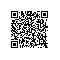# opencv笔记4：模板运算和常见滤波操作

## 模板运算

g(x,y)=function(f(x,y), template)

## 平滑滤波

### 线性平滑滤波

opencv现在有3个线性平滑滤波器：方框滤波、均值滤波、高斯滤波

### 非线性平滑滤波

opencv现在有2个非线性平滑滤波：中值滤波和双边滤波

#include <iostream>
#include <opencv2/opencv.hpp>

using namespace std;
using namespace cv;

int main(){
//【1】定义原图像
//【2】调用模糊函数
//【3】显示结果

//【1】定义原图像
Mat meanBlurImage, medianBlurImage;

//【2】调用模糊函数
blur(srcImage, meanBlurImage, Size(3,3));
medianBlur(srcImage, medianBlurImage, 3);

//【3】显示结果
imshow("原图", srcImage);
imshow("均值滤波", meanBlurImage);
imshow("中值滤波", medianBlurImage);
while(waitKey(1)!='q'){}
destroyAllWindows();

return 0;
}## 锐化滤波

### 非线性锐化滤波

#### 梯度锐化

f'(x) = f(i,j)-f(i+1,j)
f'(y) = f(i,j)-f(i,j+1)

f'(x) = f(i,j)-f(i+1, j+1)
f'(y) = f(i+1,j ) - f(i, j+1) 

x、y两个方向上差分的结果，可以通过距离公式和在一起，用来表示最后的计算结果，比如采用水平垂直差分+曼哈顿距离公式，有：

g(i,j)=|f(i,j)-f(i+1,j)|+|f(i,j)-f(i,j+1)|

g(i,j)=sqrt( (f(i,j)-f(i+1,j+1))^2 + (f(i+1,j)-f(i,j+1)^2 )

g(i,j)=|(f(i,j)-f(i+1,j+1)| + |(f(i+1,j)-f(i,j+1)|

Sx=[1  0]
[0 -1]

Sy=[0 -1]
[1  0]

Gx=Sx * A   #卷积操作
Gy=Sy * A   #卷积操作

#### Prewitt算子

   [-1 0 1]
Sx=[-1 0 1]
[-1 0 1]

[ 1  1  1]
Sy=[ 0  0  0]
[-1 -1 -1]

#### Soble和Canny

Sobel算子用来计算图像的导数，目的是获得图像边缘。因此，它常常被用于边缘检测。Canny算法是比Sobel更完整的边缘检测算法，包括了预处理（高斯滤波）、后处理（阈值法去除非边缘点）。

Sobel算子，一般取模板:

   [-1 0 1]
Sx=[-2 0 2]
[-1 0 1]

[ 1  2  1]
Sy=[ 0  0  0]
[-1 -2 -1]

Canny是指Canny边缘检测算法，其步骤包括：

1. 滤波：读取灰度图像后，使用高斯滤波做平滑处理（去噪）
2. 增强：用一阶偏导的有限差分来计算梯度的幅值和方向（锐化）。这一步可以使用Roberts、Sobel、Prewitt等算子,或者说，使用类似Sobel滤波器的滤波步骤。
3. 检测：使用阈值法将非边缘点去除，获得真正的边缘点
如此看来，Canny边缘检测是模板操作的综合应用了，既有平滑处理，也有锐化处理。

Gx(x,y)=Sx * A   #卷积操作
Gy(x,y)=Sy * A   #卷积操作
G(x,y)=sqrt(Gx^2+Gy^2)   #梯度幅值
theta=arctan(Gy(x,y)/Gx(x,y))   #梯度幅角

P.S.:opencv中的Sobel函数，如果指定的模板规格为3x3，按照前面的模板矩阵，会产生明显的不精确的结果。通过调用Scharr算子的模板，效果会好些：

   [-3  0  3]
Sx=[-10 0 10]
[-3  0  3]

[-3 -10 -3]
Sy=[ 0  0   0]
[-3 -10 -3]

### 线性锐化滤波

#### 拉普拉斯算子

g(i,j)=f''(x)+f''(y)

f''(x) = 2f(i,j)-f(i+1,j)-f(i-1,j)
f''(y) = 2f(i,j)-f(i,j+1)-f(i,j-1)
g(i,j)=4f(i,j)-f(i+1,j)-f(i-1,j)-f(i,j+1)-f(i,j-1)

 0 -1  0
-1  4 -1
0 -1  0

-1 -1 -1
-1  8 -1
-1 -1 -1

#### 高频提升滤波

h(x,y) = A*f(x,y)-g(x,y) = (A-1)*f(x,y)+(f(x,y)-g(x,y)) = (A-1)*f(x,y) + mask(x,y)

## ref

RachealZhang:双边滤波器的原理及实现

MrMystery:图像增强-图像锐化使用钉钉扫一扫加入圈子
+ 订阅